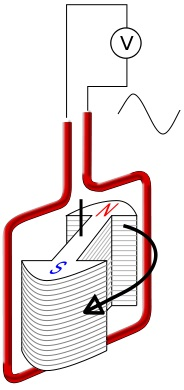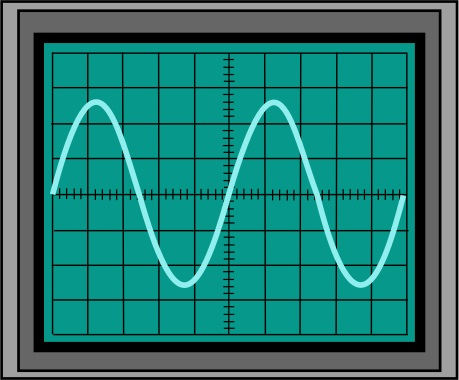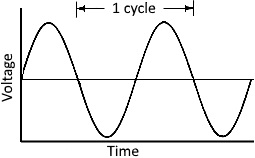#Alternating Current by Stephen Bucaro

With DC (Direct Current), electric current flows in only one direction. With AC (Alternating Current), current direction reverses periodically.

Whenever a conductor is placed in a varying magnetic field an EMF (Electromotive Force) gets induced across the conductor. If the conductor is in a circuit, this force causes current to flow in the circuit. The magnetic field can be varied by moving a magnet when near the conductor, by moving a conductor near a magnet, or by rotating a magnet while near a conductor. This is the working principle behind electric generators, electric motors, transformers, and coils.An EMF (voltage) is created in a generator by rotating a magnet near a conductor. This voltage reverses polarity when poles of opposite polarity move past the conductor.An oscilloscope is an electronic test equipment that displays, on a screen, voltage as a function of time. The point of the waveform in the vertical axis represents the voltage. The point of the waveform along the horizontal axis represents the time. The oscilloscope screen shown above displays the sign wave output of an AC generator. The center horizontal line with the tick marks is zero voltage.

The AC waveform starts out at zero volts. The voltage increases with time until it reaches its positive peak. At its peak the voltage reverses direction and decreases until it reaches its negative peak voltage. At the negative peak the voltage again reverses direction and increases until it returns to zero volts. This represents one cycle. The voltage continues to increase, starting another cycle.A cycle is defined by the points at which the waveform reaches the same voltage and the voltage going in the same direction as it was at the closest previous time. The frequency of the signal is defined by the number of cycles that occur within one second. This unit is frequently referred to as hertz (symbol Hz) after Heinrich Rudolf Hertz, the physicist who proved the existence of the electromagnetic waves. The wavelength is the with of one cycle (in meters).

Sign waves are important because of their connection with nature and mathematics. Sign waves, circles, and triangles form the basic of trigonometry, which allows us to calculate many properties of electronic circuits as well as nature and the universe.